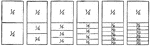### Fraction Comparisons

Rectangles comparing fraction values to one half: 1/4, 1/6, 1/8, 1/10, and 1/12.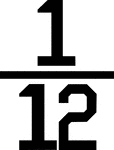### Numerical fraction 1/12

Numerical fraction 1/12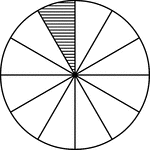### Fraction Pie Divided into Twelfths

A circle divided into twelfths with one twelfth shaded.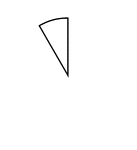### One Twelfth of a Fraction Pie

One twelfth of a circle.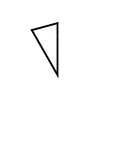### Fractions of 12-sided Polygon

1/12 of a 12 sided polygon